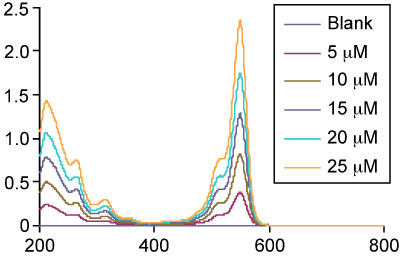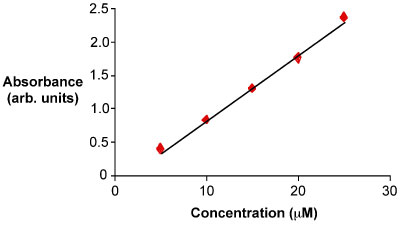# Measuring the concentration

LevelBasic

The relation of absorbance to concentration is given by Lambert-Beer's law (Beer's law): A = ɛlc
(where A is absorbance (unitless), ɛ (epsilon) is molar absorption coefficient (or molar absorption constant) of the analyte for a certain wavelength (l⋅mol-1⋅cm-1), l is path length (cm) through your cuvette and c is the concentration of the analyte (mol⋅l-1).)

• For an accurate determination of the concentration of analyte in your sample prepare at least five different solutions with known concentrations of the analyte for a standard series, this will become the calibration curve.
• It is preferred that the concentration of your analyte in your sample is somewhere in the middle of your calibration curve
• For an accurate measurement the maximum difference between the highest and the lowest known concentration of your calibration curve may only vary a factor of 10, the smaller the difference the better.
• You record spectra of a blank and the series of standard solutions. A blank contains everything that is also in your standard series of solutions except for the analyte.
• Choose the wavelength of the peak with the highest absorbance in the spectrum. Construct a calibration curve with the absorbance at this wavelength plotted against the different concentrations (figure 6.1). Fit these points with a straight line, preferably with the method of linear least squares.
• Record a spectrum of the sample and interpolate the peak intensity with the concentration, Fill in the absorbance of your sample at the line fitted through those five points, and you’ll find the concentration.
• Demo of how to calculate a concentration from a series of measuments(4:58 mins):

How to generate a standard curve, and use that standard curve to determine the concentration of an unknown solution, titled "Determining the Concentration of an Unknown Sample Using the Standard Curve Excel 2010" (10:58 mins):

Examples of a calculation with Beer's law:
1. A solution of 1 mg paracetamol in 100 ml methanol (MeOH) is measured with UV-Vis.

The intensity of the light through a blank solution of MeOH (I0) is 100% by definition.
The measured intensity of sample solution (I) is 13.18%

Formula: A = - log10T = -log10 (I/I0).
Known values: I0 = 100%
I = 13.18 %
A = -log10 (13.18/100)
A = -log10 (0.1318)
A = -(-0.880)
A = 0.880

2. A solution of 1 mg paracetamol (MW=151.17 g/mol) in 100 ml MeOH (c) is measured in a 10 mm cuvet (l) with UV-Vis.
The absorption maximum of paracetamol in MeOH is at 247nm with a molar absorption coefficient of 12850 l⋅mol-1⋅cm-1 (ɛ).
Formula: A = ɛlc
Known values: ɛ = 12850
l = 10 mm = 1 cm
c = 1 mg/100ml = 10mg/l = 0.01g/l
c = 0.01 × 1/151.17 = 6.61⋅10-5 mol/l
A = 12850 × 1 × 6.61⋅10-5
A = 0.849

For a theoretical explanation on the derivation of the Beer’s law, click this link.An example of the linear correlation – a straight line - between the concentration of the analyte and the absorbance.

A whiteboard presentation on the calibration curve and how to find an unknown concentration (13:35 mins):

Summary of tips and tricks from the experts We suggest that at this stage you do a virtual UV-Vis measurement. Try this nice java-applet from the website of the chemistry department of the Davidson College in North Carolina, click here[VirtMeas].